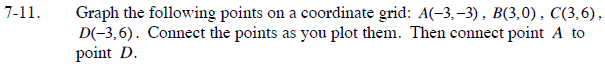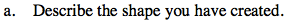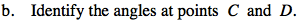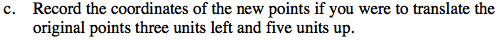### Home > CC3 > Chapter 7 > Lesson 7.1.1 > Problem7-11

7-11.
1. Graph the following points on a coordinate grid: A(−3, −3), B(3, 0), C(3, 6), D(−3, 6). Connect the points as you plot them. Then connect point A to point D. 7-11 HW eTool (Desmos). Homework Help ✎

1. Describe the shape you have created.

2. Identify the angles at points C and D.

3. Record the coordinates of the new points if you were to translate the original points three units left and five units up.There is only one pair of parallel sides.Notice that lines BC and AD are perpendicular to line CD.Subtract 3 from each x-coordinate and add 5 to each y-coordinate. For example A(−3, −3) will become A'(−6, 2).

Use the eTool below to graph the points.
Click the link at right for the full version of the eTool: CC3 7-11 HW eTool